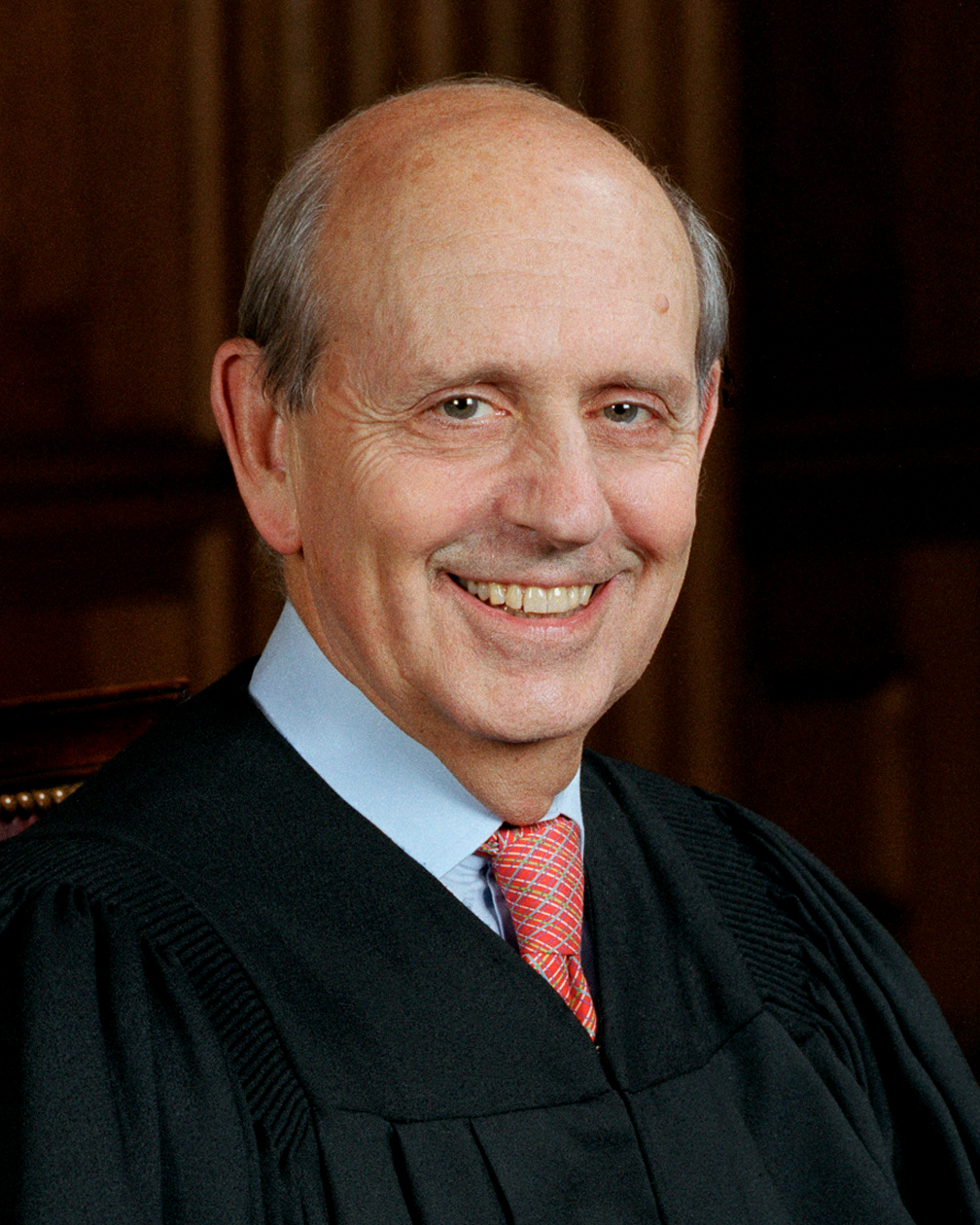Justice Breyer is a Stanford Alum

In the Supreme Court case: Berghuis v. Smith, the Supreme Court (of the US) discussed the question: "If a group is underrepresented in a jury pool, how do you tell?"

Justice Breyer [Stanford Alum] opened the questioning by invoking the binomial theorem.  He hypothesized a scenario involving “an urn with a thousand balls, and sixty are red, and nine hundred forty are green, and then you select them at random… twelve at a time.”  According to Justice Breyer and the binomial theorem, if the red balls were black jurors then “you would expect… something like a third to a half of juries would have at least one black person” on them.

Note: What is missing in this conversation is the power of diverse backgrounds when making difficult decisions.

## Explination:

Technically, since jurors are selected without replacement, you should represent the number of under-representative jurors as being a Hyper Geometric Random Variable (a random variable we don't look at explicitely in CS109) st

\begin{align*}X \sim \text{HypGeo}(n=12, N = 1000, m = 60)\end{align*}

\begin{align*} P(X \geq 1) &= 1 - P(X = 0) \\ &= 1 - \frac{ {60 \choose 0}{940 \choose 12} }{1000 \choose 12} \\ &\approx 0.5261 \end{align*}

However Justic Breyer made his case by citing a Binomial distribution. This isn't a perfect use of binomial, because the binomial assumes that each experiment has equal likelihood ($p$) of success. Because the jurors are selected without replacement, the probability of getting a minority juror changes slightly after each selection (and depending on what the selection was). However, as we will see, because the probabilities don't change too much the binomial distribution is not too far off.

\begin{align*} X \sim \text{Binomial}(n=12, p = 60/1000) \end{align*}

\begin{align*} P(X \geq 1) &= 1 - P(X = 0) \\ &= 1 - {60 \choose 0}(1- 0.06)^{12} \\ &\approx 0.5241 \end{align*}